Question

# When 60.0 mL of a 0.400 M solution of HNO3 (aq) is combined with 60.0 mL...

When 60.0 mL of a 0.400 M solution of HNO3 (aq) is combined with 60.0 mL of a 0.400 M solution of NaOH(aq) in a coffee-cup calorimeter, the final temperature of the solution is measured to be 26.6 ˚C. The initial temperature of the solutions is 24.0 ˚C. Calculate qrxn in joules, assuming the specific heat capacity of the final solution is 3.90 J·g–1·˚C–1, the density of the final solution is 1.04 g/mL, and the calorimeter constant is 45 J/oC. (Hint: start with qrxn + qsoln + qcal = 0.)

The balanced chemical equation for this reaction is:

HNO3 (aq) + NaOH(aq) → H2O(l) + NaNO3 (aq)

Q(rxn) = - ( Q(sol) + Q (cal))

Total volume = 60.0 + 60.0 = 120.0 ml

Mass = volume * density

= 120.0 * 1.04

= 124.8 grams

Q ( sol ) = mass * specific heat * ∆T

= 124.8 * 3.90 * ( 26.6 - 24.0 )

= 1265.472 J

Q ( cal ) = C(cal) * ∆T

= 45 * ( 26.6 - 24.0)

= 117 J

Q total = 1265.472 + 117

= 1382.472 J

Qrxn = - 1382.472 J

If you have any questions please comment

If you satisfied with the solution please rate it thanks

#### Earn Coins

Coins can be redeemed for fabulous gifts.

Similar Homework Help Questions
• ### Reaction 1: Sodium hydroxide + Hydrochloric acid Volume (mL) of 2.0 M HCl(aq) used 25.2 Initial...

Reaction 1: Sodium hydroxide + Hydrochloric acid Volume (mL) of 2.0 M HCl(aq) used 25.2 Initial temperature (°C) of the 2.0 M HCl(aq) 21.63 Volume (mL) of 2.0 M NaOH(aq) used 24.8 Maximum temperature (°C) of the 2.0 M NaOH(aq) 21.96 NaOH and HCl final temperature: 35.14 degrees C Determine the Energy (J) absorbed (+) or released (-) by the solution (qsoln). Determine the Energy (J) absorbed (+) or released (-) by the calorimeter (qcal). Determine the Energy (J) absorbed...

• ### Consider the reaction between sulfuric acid and sodium hydroxide: H2SO4(aq) + 2 NaOH(aq) → Na2SO4(aq) +...

Consider the reaction between sulfuric acid and sodium hydroxide: H2SO4(aq) + 2 NaOH(aq) → Na2SO4(aq) + 2 H2O(l)                  ΔHrxn = -111 kJ 125 mL of 0.400 M H2SO4(aq) and 125 mL of 0.400 M NaOH(aq) is mixed in a coffee cup calorimeter. Calculate qrxn. The reaction goes to completion.

• ### Suppose you mix 200.0 mL of 0.200 M RbOH(aq) with 100.0 mL of 0.400 M HBr...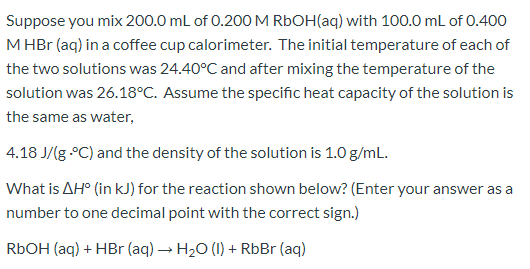Suppose you mix 200.0 mL of 0.200 M RbOH(aq) with 100.0 mL of 0.400 M HBr (aq) in a coffee cup calorimeter. The initial temperature of each of the two solutions was 24.40°C and after mixing the temperature of the solution was 26.18°C. Assume the specific heat capacity of the solution is the same as water, 4.18J/(g- C) and the density of the solution is 1.0 g/mL. What is AH° (in kJ) for the reaction shown below? (Enter your answer...

• ### HCl(aq) + NaOH(aq) → NaCl(aq) + H2O(1) 100.0 mL of 0.500 M HCl is mixed with...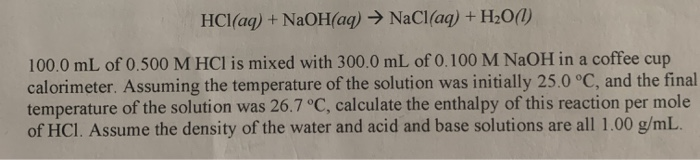HCl(aq) + NaOH(aq) → NaCl(aq) + H2O(1) 100.0 mL of 0.500 M HCl is mixed with 300.0 mL of 0.100 M NaOH in a coffee cup calorimeter. Assuming the temperature of the solution was initially 25.0 °C, and the final temperature of the solution was 26.7 °C, calculate the enthalpy of this reaction per mole of HCl. Assume the density of the water and acid and base solutions are all 1.00 g/mL.

• ### 100.0 mL of 0.400 M aqueous NaOH and 50.00 mL of 0.400 M aqueous H2SO4, each...

100.0 mL of 0.400 M aqueous NaOH and 50.00 mL of 0.400 M aqueous H2SO4, each at 24.00°C, were mixed, see equation: 2NaOH(aq) + H2SO4(aq) → Na2SO4(aq) + 2H2O(l) The final temperature achieved by the solution was 26.65 °C. Neglect the heat capacity of the cup and the thermometer, and assume that the solution of products has a density of exactly 1.00 g/mL and specific heat capacity of 4.18 J/(g•C) How much heat did the reaction release?

• ### Page 36 When 50.0 mL of 1.00 M HCI(aq) and 50.0 mL of 1.00 M NaOH(aq),...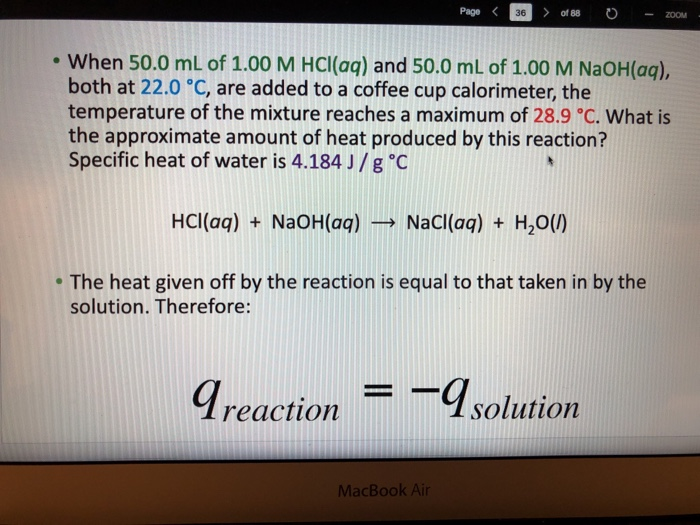Page 36 When 50.0 mL of 1.00 M HCI(aq) and 50.0 mL of 1.00 M NaOH(aq), both at 22.0 °C, are added to a coffee cup calorimeter, the temperature of the mixture reaches a maximum of 28.9 C. What is the approximate amount of heat produced by this reaction? Specific heat of water is 4.184 J/g C HCl(aq) + NaOH(aq) → Nacl(aq) + H2O() The heat given off by the reaction is equal to that taken in by the solution....

• ### If 1.85 g MgO is combined with enough 1.00 M HCl to make 100.0 mL of...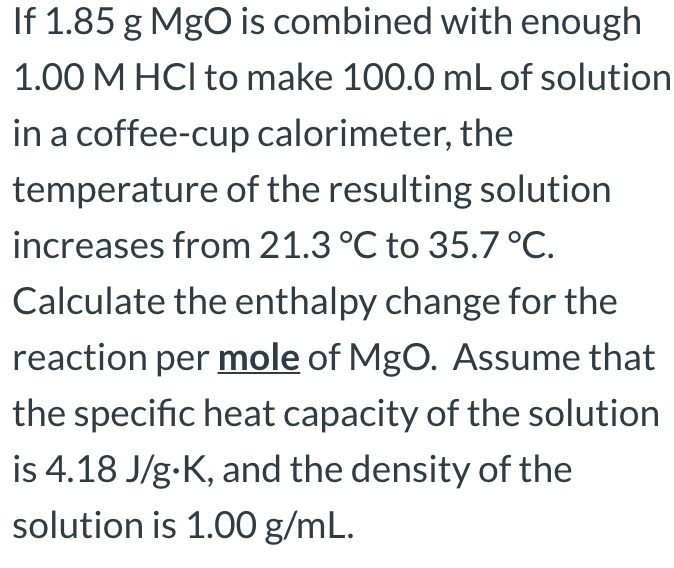If 1.85 g MgO is combined with enough 1.00 M HCl to make 100.0 mL of solution in a coffee-cup calorimeter, the temperature of the resulting solution increases from 21.3°C to 35.7 °C. Calculate the enthalpy change for the reaction per mole of Mgo. Assume that the specific heat capacity of the solution is 4.18 J/g.K, and the density of the solution is 1.00 g/mL. A 0.704 g of a pure acid, HA, is dissolved in water and an acid-base...

• ### A 100.0 mL sample of 0.400 mol L-1 HCl(aq) is added to 150.0 mL of 0.300 mol LNH3(aq) (which can be thought of as a...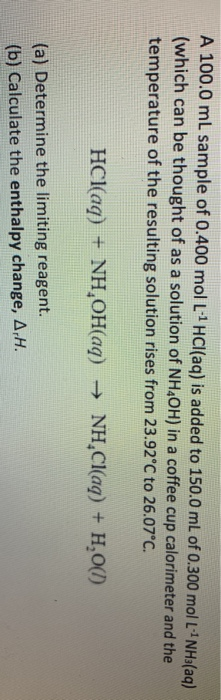A 100.0 mL sample of 0.400 mol L-1 HCl(aq) is added to 150.0 mL of 0.300 mol LNH3(aq) (which can be thought of as a solution of NH4OH) in a coffee cup calorimeter and the temperature of the resulting solution rises from 23.92°C to 26.07°C. HCl(aq) + NH,OH(aq) → NH.Cl(aq) + H2O(1) (a) Determine the limiting reagent. (b) Calculate the enthalpy change, A.H.

• ### hen 170. mL of 0.209 M NaCl(aq) and 170. mL of 0.209 M AgNO3(aq), both at...

hen 170. mL of 0.209 M NaCl(aq) and 170. mL of 0.209 M AgNO3(aq), both at 21.6°C, are mixed in a coffee cup calorimeter, the temperature of the mixture increases to 24.2°C as solid AgCl forms. NaCl(aq) + AgNO3(aq) → AgCl(s) + NaNO3(aq) This precipitation reaction produces 3.68 ✕ 103 J of heat, assuming no heat is absorbed by the calorimeter, no heat is exchanged between the calorimeter and its surroundings, and that the specific heat and density of the...

• ### When 0.615 g Mn is combined with enough hydrochloric acid to make 100.0 mL of solution in a coffee-cup calorimeter

When 0.615 g Mn is combined with enough hydrochloric acid to make 100.0 mL of solution in a coffee-cup calorimeter, all of the Mn reacts, raising the temperature of the solution from 24.0 °C to 29.4°C. Find ΔHrxn for the reaction as written. (Assume that the specific heat capacity of the solution is 4.18 J/g °C and the density is 1.00 g/mL.) Express your answer using three significant figures.